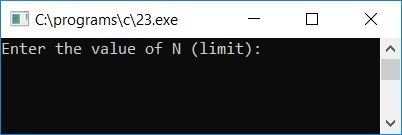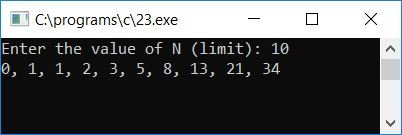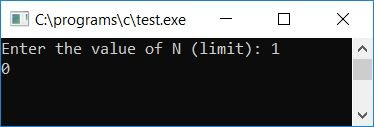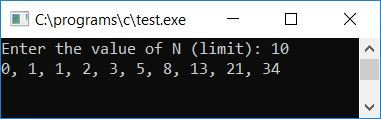# C Program to Print the Fibonacci Series

In this article, you will learn and get code for printing Fibonacci series with and without using functions. But before going through the program, let's first understand the Fibonacci series.

### What exactly is the Fibonacci Sequence?

The Fibonacci series is a series of numbers that starts at 0 and 1. Thereafter, the next number will be the sum of the previous two numbers. For example,

`0  1  1  2  3  5  8  13  21.....`

That is, the first and second numbers are 0 and 1, thereafter.

• The third number is equal to the sum of the first and second numbers. Therefore, the third number is 0+1 (which equals 1).
• fourthNumber = third + second. Therefore, the fourth number is 1+1 (which equals 2).
• fifthNumber = fourth + third. Therefore, the fifth number is 2+1 (which equals 3).
• and so on.

Now let's move on and implement it in a C program.

## Fibonacci Sequence without Function

The question is, "Write a program in C to print Fibonacci series up to N terms." The user must choose the value of N (at run-time). The answer to this question is:

```#include<stdio.h>
#include<conio.h>
int main()
{
int a=0, b=1, c, N, temp, i;
printf("Enter the value of N (limit): ");
scanf("%d", &N);
for(i=1; i<=N; i++)
{
if(i==1)
c = 0;
else if(i==2 || i==3)
c = 1;
else
{
temp = a;
a = b;
b = c;
c = a+b;
}
if(i==N)
printf("%d", c);
else
printf("%d, ", c);
}
getch();
return 0;
}```

Because the program was written in the Code::Blocks IDE, you will see the following output after a successful build and run:Provide the value of N (limit), which is how many terms of the Fibonacci series you want to print:#### Program Explained

• Initialize the first number, say a, with 0 and the second number, say b, with 1 at the beginning of the program.
• Take the limit value and say N.
• Create a for loop to run from 1 to N (the limit provided by the user at run-time).
• Inside the for loop:
• Initialize 0 to the third number, saying c if i is equal to 1.
• Initialize 1 to the third number, say c, if i is equal to 2 or 3. Otherwise, initialize the sum of the first and second number, say a and b, to the third number, say c.
• Before initializing a+b to c, make sure to initialize b to a and c to b using the temp variable.
• Then print the value of the third number, say c.

## Print the Fibonacci series using a user-defined function

Here is another program that works the same as the previous one. The only difference is that this program uses a user-defined function printFibo() to print the Fibonacci series:

```#include<stdio.h>
#include<conio.h>
void printFibo(int);
int main()
{
int N;
printf("Enter the value of N (limit): ");
scanf("%d", &N);
printFibo(N);
getch();
return 0;
}
void printFibo(int n)
{
int i, a=1, b=1, c, temp;
for(i=1; i<=n; i++)
{
if(i==1)
c = 0;
else if(i==2 || i==3)
c = 1;
else
{
temp = a;
a = b;
b = c;
c = a+b;
}
if(i==n)
printf("%d", c);
else
printf("%d, ", c);
}
}```

Here is the final snapshot of the first sample run:Here is the final snapshot of another sample run:## Using a Function to Return the Fibonacci Sequence

Let's modify the previous program so that it will also print the Fibonacci series but use the function that returns values. Here we have created a function in such a way that it will return each and every term of the Fibonacci series one by one:

```#include<stdio.h>
#include<conio.h>
int getFibo(int);
int main()
{
int N, i, fib;
printf("Enter the value of N (limit): ");
scanf("%d", &N);
for(i=1; i<=N; i++)
{
fib = getFibo(i);
if(i==N)
printf("%d", fib);
else
printf("%d, ", fib);
}
getch();
return 0;
}
int getFibo(int i)
{
static int a=0, b=1, c;
int temp;
if(i==1)
c = 0;
else if(i==2 || i==3)
c = 1;
else
{
temp = a;
a = b;
b = c;
c = a+b;
}
return c;
}```

Here is the final snapshot of the sample run of the above program that was created using a function with a return value:If the user supplies the limit as 10, then 10 starting terms of the Fibonacci series will be printed at the output screen, and the function created in the above program named getFibo() will also run 10 times to return each and every term of the Fibonacci series one by one.

Here I've used a static type variable for a, b, and c to remember its previous value throughout the program.

#### The same program in different languages

C Quiz

« Previous Program Next Program »